## F.4 Modified signal under test

38.101-13GPPNRPart 1: Range 1 StandaloneRelease 17TSUser Equipment (UE) radio transmission and reception

Implicit in the definition of EVM is an assumption that the receiver is able to compensate a number of transmitter impairments.

The DFT-s-OFDM modulated signals or PRACH signal under test is modified and, in the case of DFT-s-OFDM modulated signals, decoded according to: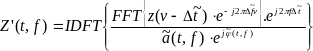where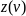is the time domain samples of the signal under test.

The CP-OFDM modulated signals or PUSCH demodulation reference signal or PUCCH data signal under test is equalised and, in the case of CP-OFDM modulated signals decoded according to: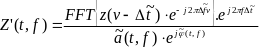where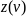is the time domain samples of the signal under test.

To minimize the error, the signal under test should be modified with respect to a set of parameters following the procedure explained below.

Notation: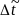is the sample timing difference between the FFT processing window in relation to nominal timing of the ideal signal.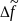is the RF frequency offset.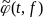is the phase response of the TX chain.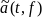is the amplitude response of the TX chain.

In the following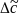represents the middle sample of the EVM window of length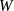(defined in the next clauses) or the last sample of the first window half if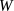is even.

The EVM analyser shall

– detect the start of each slot and estimate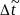and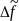,

– determine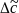so that the EVM window of length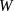is centred

– on the time interval determined by the measured cyclic prefix minus 16κ samples of the considered OFDM symbol for symbol l for subcarrier spacing configuration µ in a subframe, with l = 0 or l = 7*2^µ for normal CP, i.e. the first 16κ samples of the CP should not be taken into account for this step. In the determination of the number of excluded samples, a sampling rate of 1/Tc is assumed. If a different sampling rate is used, the number of excluded samples is scaled linearly.

– on the measured cyclic prefix of the considered OFDM symbol symbol for all other symbols for normal CP and for symbol 0 to 11 for extended CP.

– on the measured preamble cyclic prefix for the PRACH

To determine the other parameters a sample timing offset equal to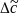is corrected from the signal under test. The EVM analyser shall then

– correct the RF frequency offset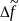for each time slot, and

– apply an FFT of appropriate size. The chosen FFT size shall ensure that in the case of an ideal signal under test, there is no measured inter-subcarrier interference.

The carrier leakage shall be removed from the evaluated signal before calculating the EVM and the in-band emissions; however, the removed relative carrier leakage power also has to satisfy the applicable requirement.

At this stage the allocated RBs shall be separated from the non-allocated RBs. In the case of PUCCH and PUSCH EVM, the signal on the non-allocated RB(s),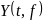, is used to evaluate the in-band emissions.

Moreover, the following procedure applies only to the signal on the allocated RB(s).

– In the case of PUCCH and PUSCH, the UL EVM analyzer shall estimate the TX chain equalizer coefficients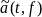and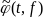used by the ZF equalizer for all subcarriers by time averaging at each signal subcarrier of the amplitude and phase of the reference and data symbols. The time-averaging length is 1 slot. This process creates an average amplitude and phase for each signal subcarrier used by the ZF equalizer. The knowledge of data modulation symbols may be required in this step because the determination of symbols by demodulation is not reliable before signal equalization.

– In the case of PRACH, the UL EVM analyzer shall estimate the TX chain coefficients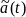and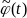used for phase and amplitude correction and are seleted so as to minimize the resulting EVM. The TX chain coefficients are not dependent on frequency, i.e.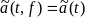and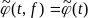. The TX chain coefficient are chosen independently for each preamble transmission and for each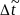.

At this stage estimates of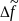,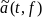,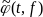and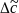are available.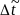is one of the extremities of the window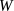, i.e.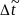can be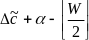or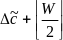, where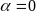if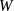is odd and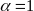if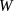is even. The EVM analyser shall then

– calculate EVMl with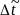set to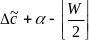,

– calculate EVMh with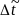set to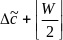.

For the EVM calculation on the symbols with a transient period when the UE signals a transient period capability (tp) of 2, 4 or 7usec, is is given below.

– calculate EVMl_tp with set to, where is 1/Tc the sampling rate

– calculate EVMh_tp with set to, where 1/Tc is the sampling rate and the CP is the cyclic prefix of the symbol on which EVM is calculated(e.g. long CP for the first symbol of the slot) in seconds

A pictorial representation of the EVM measurement windows is given in Figure F.4-1.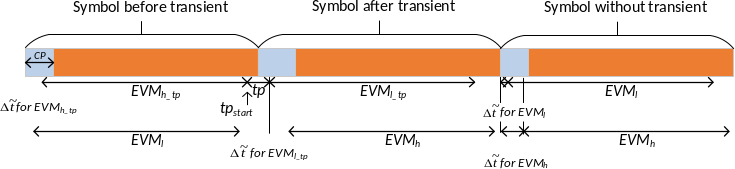Figure F.4-1 EVM measruement window